Solve for X
Relationships
Describing Associations
Identifying Slope & y-intercept
Solve for Y
100

3x =8

What is 2.66

100

What is the relationship (association) between distance traveled and gallons of gas used?

positive association

100

Describe this association.Strong, Positive

100

y= 3X + 4

What is the slope?
What is the y-intercept?

Slope: 3
y-intercept: 4

100

4y + 3x = 19

y=-3/4x+19/4

200

5x =7

What is 1.4?

200

What is the relationship (association) between hours spent watching TV and math test grade?

negative association

200

Describe this association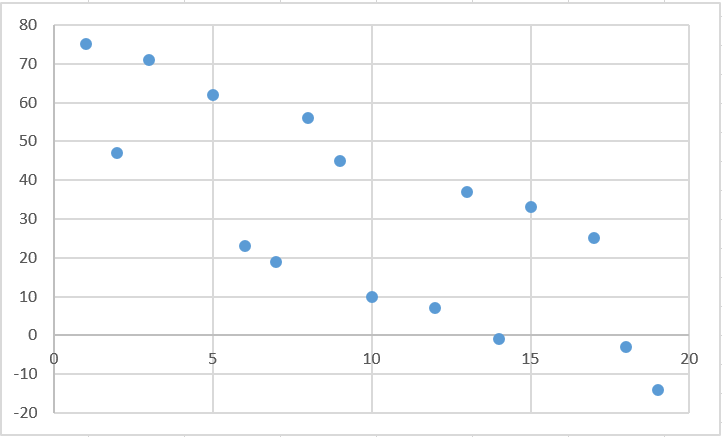Weak, Negative

200

y=6x+12

What is the slope?
What is the y-intercept?

Slope: 6
y-intercept: 12

200

6x + 3y =12

y=-2x+4

300

2x + 8y =12, when y=0

What is 6?

300

What is the association between a child's weight and math grade?

no association

300

Describe the association: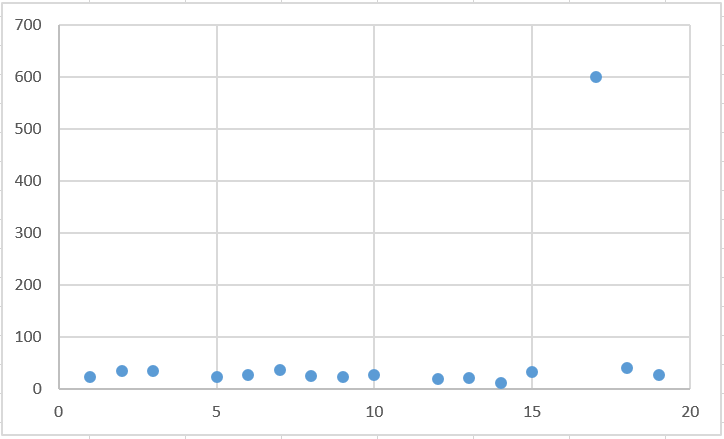No association, with an outlier

300

y=12 + 6x

What is the slope?
What is the y-intercept?

slope: 6

y-intercept: 12

300

6x + 3y =14

What is y=-2x+14/3(4.667)

400

11x + 3y =17, when y=1

What is 1.2727?

400

What is missing from the following scatterplot?A title, labels on the axes, units

400

Describe the association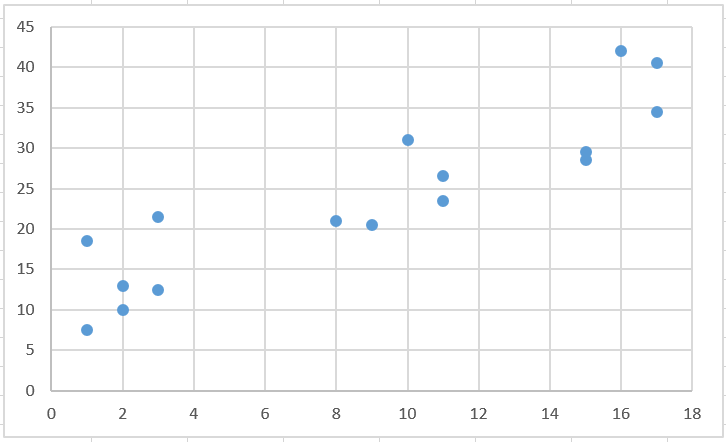Weak Positive/ or No Correlation

400

y=-6 + 7x

What is the slope?
What is the y-intercept?

Slope: 7

y-intercept: -6

400

13 x + 9 y = 12

What is y=-13/9x+12/9?

(y=-1.44x+1.33)

500

6x + 4y =21, when y=3

What is 1.5?

500

Would this be a positive association or a negative association? (Hint: Imagine how it would look in a scatter plot.)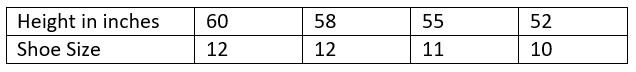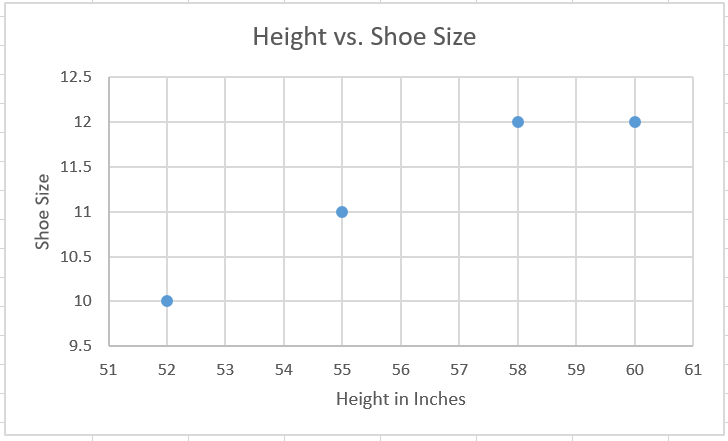positive association

500

Describe the associationStrong, Positive

500

16-2.5x = y

What is the slope?
What is the y-intercept?

slope: -2.5

y-intercept: 16

500

2x + 8 y = 144

What is y=-1/4x+18

Click to zoom